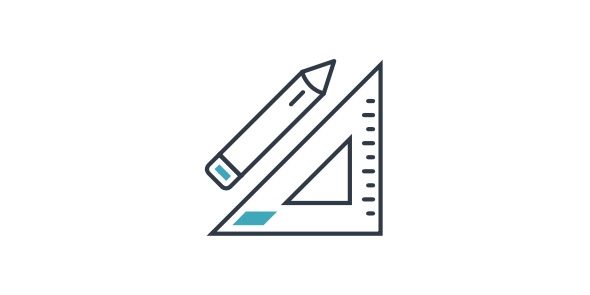# Math Exam For 3rd Grade Student

15 Questions | Total Attempts: 147SettingsDo you really know everything about 3rd grade math? Take this quiz to test yourself

• 1.
If Cody has 22 cents, what is the largest number of coins he can have?
• A.

6

• B.

5

• C.

22

• D.

4

• 2.
If we divide 17 by 5, what is our remainder?
• A.

3

• B.

5

• C.

1

• D.

2

• 3.
What is 255 - 48?
• A.

217

• B.

207

• C.

197

• D.

187

• 4.
What is 250 rounded to the nearest 100?
• A.

300

• B.

400

• C.

200

• D.

100

• 5.
What is 0 x 6?
• A.

1

• B.

6-Jan

• C.

0

• D.

6

• 6.
Which number is largest?
• A.

351

• B.

976

• C.

319

• D.

721

• 7.
If Lisa can ride her bike 5 miles in 30 minutes, how far can she ride in 1 hour?
• A.

2.5 Miles

• B.

5 Miles

• C.

15 Miles

• D.

10 Miles

• 8.
What is the remainder when 2638 is divided by 24?
• A.

24

• B.

23

• C.

25

• D.

22

• 9.
How many groups of 4 do you need to make 12?
• A.

4

• B.

3

• C.

2

• D.

12

• 10.
How do you write 7 hundreds + 8 tens + 9 ones in standard form?
• A.

789

• B.

897

• C.

798

• D.

987

• 11.
What is 33 + 6 + 20?
• A.

59

• B.

49

• C.

79

• D.

69

• 12.
What number can be added to any number to get your same number back?
• A.

10

• B.

0

• C.

1

• D.

-1

• 13.
What is 479 rounded to the nearest 100?
• A.

400

• B.

600

• C.

500

• D.

300

• 14.
What is 14 x 6?
• A.

64

• B.

74

• C.

94

• D.

84

• 15.
What is 6001 - 4569?
• A.

1332

• B.

1122

• C.

1432

• D.

1532

Related Topics